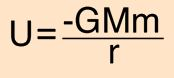Gravitational potential energy

the gravitational potential at a location is equal to the work (energy transferred) per unit mass that would be done by the force of gravity if an object were moved from its location in space to a fixed reference location. It isanalogous to the electric potential with mass playing the role of charge. The reference location, where the potential is zero, is by convention infinitely far away from any mass, resulting in a negative potential at any finite distance.where G is the gravitation constant, M is the mass of the attracting body, and r is the distance between their centers.

Gravitational potential energy called “GPE” is a measure of how far an object can fall. The higher up it is , the further it can fall and the more GPE it has

GPE also depends on the object’s weight

GPE = mass * gravity * height

GPE = m * g * h